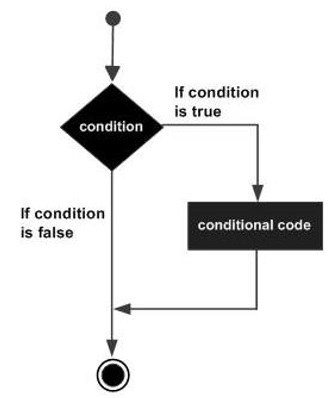# Groovy - If Statement

The first decision making statement is the if statement. The general form of this statement is −

```if(condition) {
statement #1
statement #2
...
}
```

The general working of this statement is that first a condition is evaluated in the if statement. If the condition is true, it then executes the statements. The following diagram shows the flow of the if statement.Following is an example of a if/else statement −

```class Example {
static void main(String[] args) {
// Initializing a local variable
int a = 2

//Check for the boolean condition
if (a<100) {
//If the condition is true print the following statement
println("The value is less than 100");
}
}
}
```

In the above example, we are first initializing a variable to a value of 2. We are then evaluating the value of the variable and then deciding whether the println statement should be executed. The output of the above code would be −

```The value is less than 100
```
groovy_decision_making.htm